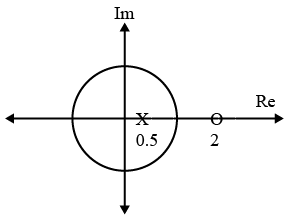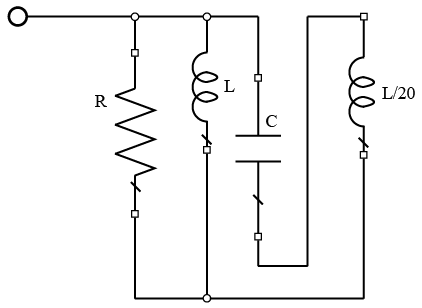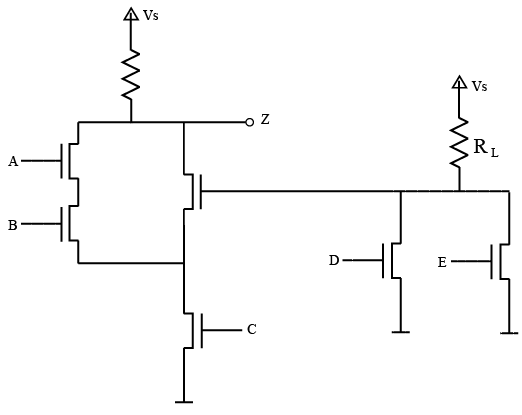# ISRO Scientist or Engineer Electronics 2010

Instructions

For the following questions answer them individually

Question 1

# An LTI system has the input signal x(n). Which of the following sequence of operations is most appropriate to get output $$y(n) = x \left(n - \frac{M}{L}\right)$$.Question 2

# Consider a low pass random process with a white noise power spectral density$$S_{x} (\omega)=\frac{N}{2}$$ where $$-2 \pi B \leq \omega \leq 2 \pi B$$                     0 elsewhereThe Auto-correlation function $$R_{x}(\tau)$$ isQuestion 3

# Pole-zero plot of a digital filter is shown below, what is the type of filter?Question 4

# Determine the DTFT of the sequence $$y[n] = (n + 1) \alpha^{n} u[n], \mid \alpha \mid < 1.u[n]$$ is unit step sequenceQuestion 5

# The function f(t) has the Fourier transform g$$(\omega)$$. The Fourier transform of g(t) isQuestion 6

# The purpose of Design for Test (DFT) process in ASIC design flow isQuestion 7

# The coupling between the two inductors is increased from zero in the circuit shown.' Which of the following statements is true?Question 8

# Write a Boolean expression for Z in terms of A, B, C, D and E. You need not simplify the expression.Question 9

# Aparallel plate capacitor of 100 pf having an air dielectric is charged to 10 kilovolts. It is then electrically isolated. The plates are pulled away from each other until the distance is ten times more than before. Estimate the energy neededto pull the plates.-Question 10

# How could Schroedinger’s equation be relevant in the practical design of a CathodeRay Tube?OR